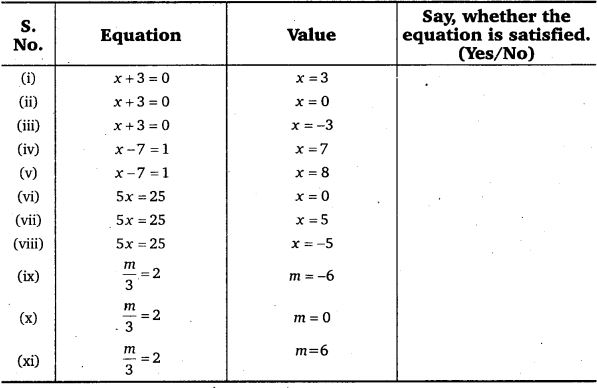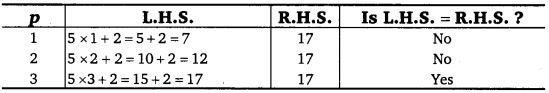# Class 7 Maths NCERT Solutions for Chapter – 4 Simple Equations EX – 4.1

## Simple Equations

Question 1.
Complete the last column of the table.Solution:

Question 2.
Check whether the value given in the brackets is a solution to the given equation or not :
(a)
n + 5 = 19 (n = 1)
(b) 7n + 5 = 19 (w = -2)
(c) 7n + 5 = 19 (n = 2)
(d) 4p – 3 = 13(p = 1)
(e) 4p-3 = 13(p = -4)
(f) 4p – 3 = 13 (p = 0)
Solution:

Question 3.
Solve the following equations by trial and error method :
(i)
5p + 2 = 17 .
(ii) 3m – 14 = 4

Solution:
(i) Let us evaluate the L.H.S. and R.H.S. of the given equation for some values of p and continue to given new values till the L.H.S. becomes equal to the R.H.S.
The given equation is 5p + 2 = 17. We have,
L.H.S. = 5p+ 2 and R.H.S. = 17Clearly, L.H.S. = R.H.S. for p = 3. Hence, p = 3 is the solution of the given equation.

(ii) Let us evaluate the L.H.S. and R.H.S. of the given equation for some values of m and continue to given new values till the L.H.S. becomes equal to the R.H.S.
The given equation is 3m – 14 = 4, that is, 14 subtracted from 3 times m gives 4. So, we substitute values which gives 3m > 14. We have, L.H.S. = 3m – 14 and R.H.S. = 4Clearly, L.H.S. = R.H.S. for m = 6. Hence, m = 6 is the solution of the given equation.

Question 4.
Write equations for the following statements :

1. The sum of numbers x and 4 is 9.
2. 2 subtracted from y is 8.
3. Ten times a is 70.
4. The number b divided by 5 gives 6.
5. Three-fourth of t is 15.
6. Seven times m plus 7 gets you 77.
7. One fourth of a number x minus 4 gives 4.
8. If you take away 6 from 6 times y, you get 60.
9. If you add 3 to one third of z, you get 30.

Solution:
The equations for the given statements are :

1. x + 4 = 9
2. y – 2 = 8
3. 10 a = 70
4. b ÷ 5 = 6
5.$\frac { 3 }{ 4 }$ × t = 15
6. 7m + 7 = 77
7.$\frac { 1 }{ 4 }$ × x – 4 = 4
8. 6y – 6 = 60
9.$\frac { 1 }{ 3 }$ × z + 3 = 30

Question 5.
Write the following equations in statement forms :

1. p + 4 = 15
2. m – 7 = 3
3. 2m = 7
4.$\frac { m }{ 5 }$ = 3
5.$\frac { 3m }{ 5 }$= 6
6. 3p + 4 = 25
7. 4p – 2 = 18
8.$\frac { p }{ 2 }$ + 2 = 8

Solution:
The statements for the given equations are :

1. The sum of numbers p and 4 is 15.
2. The difference of m and 7 is 3.
3. Two times m is 7.
4. The number m divided by 5 gives 3.
5. Three time’s m divided by 5 gives 6.
6. Three times p plus 4 gives 25.
7. Four times p minus 2 gives 18.
8. p divided by 2 plus 2 gives 8.

Question 6.
Set up an equation in the following cases :
(i)
Irfan says that he has 7 marbles more than five times the marbles Parxnit has. Irfan has 37 marbles. (Take m to be the number of Permits marbles.)

(ii) Laxmi’s father is 49 years old. He is 4 years older than three times Laxmi’s age. (Take Laxmi’s age to be y years.)

(iii) The teacher tells the class that the highest marks obtained by a student in her class is twice the lowest marks plus 7. The highest score is 87. (Take the lowest score to be l.)

(iv) In an isosceles triangle, the vertex angle is twice either base angle. (Let the base angle be b in degrees. Remember that the sum of angles of a triangle is 180 degrees).

Solution:
(i) Let the number of marbles with Parmit be m.
Then, 7 added to 5 times mis 5m + 7
It is given that 7 marbles more than five times the marble is 37. Thus, the equation obtained is 5m + 7 = 37.

(ii) Let Laxmi’s age be y years. Then, 4 added to 3 times y is 3y + 4
It is given that the father is 4 years older than 3 times Laxmi’s age. His age is 49years.
Then, we have the following equation : 3y + 4 = 49

(iii) Let the lowest marks be l. Then, twice the lowest marks plus 7 is 2l +7
It is given that, the highest marks 87 obtained by a student is twice the lowest marks plus 7.
So, we have the following equation : 2l + 7 = 87

(iv) Let the base angle be b. Then, the vertex angle = 2b.
Since, sum of the angles of a triangle is 180°
∴ b + b + 2b = 180°
⇒ 4b = 180°
which is the required.equation.